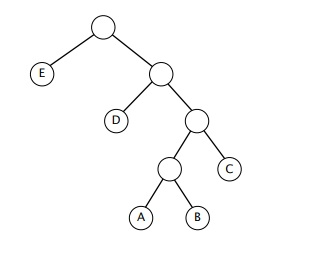### DATA COMPRESSION STUDY GUIDE

Terminology and Basics

• Why compress? To save space and time.
• How does compression work? Compression takes advantage of structure within data.
• What sort of data does not compress well? Random data.
• Lossy compression can further reduce file sizes by throwing away unimportant information.
• What is the compression ratio?
• Why can no compression algorithm possibly compress all bit streams?
• What fraction of bitstreams can be compressed in half by a general-purpose algorithm?
Run-length coding
• Takes advantage of repeated binary digits.
• How do you handle runs of length more than 2M?
Huffman coding
• Basic idea: Variable length codewords to represent fixed length characters. More common characters are represented by shorter codewords.
• What is a prefix-free code? Why is it important that Huffman coding use a prefix-free code? Would encoding work with a non prefix-free code? Would decoding work?
• Why is it necessary to transmit the coding trie? Why don't we have to do something similar with run length encoding or LZW?
• Why do we typically use an array for encoding and a trie for decoding?
• You do not need to know the specifics of the binary representaiton of the Huffman trie. However, you should conceptually understand the idea of transmitting/reading the trie using an preorder traversal.
LZW
• Basic idea: Fixed length codewords to represent variable length strings. More common characters are represented by shorter codewords.
• Why do we typically use a trie for encoding and an array for decoding?
• How do you handle the 'strange' case (where a codeword is seemingly not in the table during decoding)?

### Recommended Problems

#### C level

1. Decode each of the following LZW-encoded messages or explain briefly why it is not a valid LZW-encoded message. (Recall that codeword 80 is reserved to signify end of file.)
```41 42 43 44 80
42 41 4E 82 41 80
42 41 83 80
41 42 81 82 80
41 42 81 83 80
42 41 4E 44 41 4E 41 80
```
2. Fall 2012 Final, #12 (Huffman)
3. Spring 2012 Final, #10 (BW)
4. Textbook 5.5.3

#### B level

1. Consider the following Huffman trie of a message over the 5-character alphabet {A, B, C, D, E}:Identify each statement with the best matching description (below tagged with letters A to D).
---- The frequency of A is strictly less than the frequency of B.
---- The frequency of C is greater than or equal to the frequency of A.
---- The frequency of D is strictly greater than the frequency of A.
---- The frequency of D is greater than or equal to that of A, B, and C combined.
---- The frequency of E is strictly less than that of
```    A, B, and C combined.
A. True for all messages.
B. False for all messages.
C. Depends on the message.
```